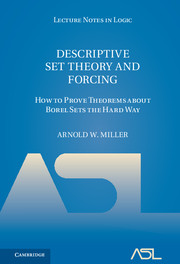# Lecture Notes in Logic, 4

## Descriptive Set Theory and Forcing: How to Prove Theorems about Borel Sets the Hard Way

Arnold W. MillerYear: 2017
ISBN: 9781107168060

In this volume, the fourth publication in the Lecture Notes in Logic series, Miller develops the necessary features of the theory of descriptive sets in order to present a new proof of Louveau’s separation theorem for analytic sets. While some background in mathematical logic and set theory is assumed, the material is based on a graduate course given by the author at the University of Wisconsin, Madison, and is thus accessible to students and researchers alike in these areas, as well as in mathematical analysis.

1. What are the reals, anyway

Part I. On the Length of Borel Hierarchies:

1. Borel hierarchy
2. Abstract Borel hierarchies
3. Characteristic function of a sequence
4. Martin’s axiom
5. Generic Gδ
6. α-forcing
7. Boolean algebras
8. Borel order of a field of sets
9. CH and orders of separable metric spaces
10. Martin–Soloway theorem
11. Boolean algebra of order ω1
12. Luzin sets
13. Cohen real model
14. The random real model
15. Covering number of an ideal

Part II. Analytic Sets:

1. Analytic sets
2. Constructible well-orderings
3. Hereditarily countable sets
4. Schoenfield absoluteness
5. Mansfield–Soloway theorem
6. Uniformity and scales
7. Martin’s axiom and constructibility
8. Σ12 well-orderings
9. Large Π12 sets

Part III. Classical Separation Theorems:

1. Souslin–Luzin separation theorem
2. Kleen separation theorem
3. Π11 -reduction
4. Δ11 -codes

Part IV. Gandy Forcing:

1. Π11 equivalence relations
2. Borel metric spaces and lines in the plane
3. Σ11 equivalence relations
4. Louveau’s theorem
5. Proof of Louveau’s theorem
• References
• Index
• Elephant sandwiches